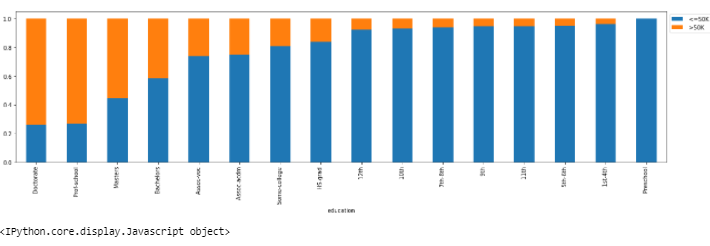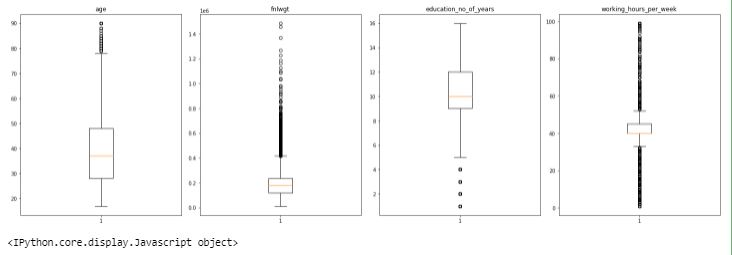Search

# Income Group Classification Using Python Machine Learning

### Dataset

The data contains characteristics of the people

• age: continuous - age of a Person

• workclass: Where does a person works - categorical -Private, Self-emp-not-inc, Self-emp-inc, Federal-gov, Local-gov, State-gov, Without-pay, Never-worked.

• fnlwgt: continuous - Weight assigned by Current Population Survey (CPS) - People with similar demographic characteristics should have similar weights since it is a feature aimed to allocate similar weights to people with similar demographic characteristics.

• education: Degree the person has - Bachelors, Some-college, 11th, HS-grad, Prof-school, Assoc-acdm, Assoc-voc, 9th, 7th-8th, 12th, Masters, 1st-4th, 10th, Doctorate, 5th-6th, Preschool.

• education-num: no. of years a person studied - continuous.

• marital-status: Married-civ-spouse, Divorced, Never-married, Separated, Widowed, Married-spouse-absent, Married-AF-spouse.

• occupation: Tech-support, Craft-repair, Other-service, Sales, Exec-managerial, Prof-specialty, Handlers-cleaners, Machine-op-inspct, Adm-clerical, Farming-fishing, Transport-moving, Priv-house-serv, Protective-serv, Armed-Forces.

• race: White, Asian-Pac-Islander, Amer-Indian-Eskimo, Other, Black.

• sex: Female, Male.

• capital-gain: Investment gain of the person other than salary - continuous

• capital-loss: Loss from investments - continuous

• hours-per-week: No. of hours a person works - continuous.

• native-country: United-States, Cambodia, England, Puerto-Rico, Canada, Germany, Outlying-US(Guam-USVI-etc), India, Japan, Greece, South, China, Cuba, Iran, Honduras, Philippines, Italy, Poland, Jamaica, Vietnam, Mexico, Portugal, Ireland, France, Dominican-Republic, Laos, Ecuador, Taiwan, Haiti, Columbia, Hungary, Guatemala, Nicaragua, Scotland, Thailand, Yugoslavia, El-Salvador, Trinidad&Tobago, Peru, Hong, Holand-Netherlands.

• salary: >50K, <=50K (dependent variable, the salary is in Dollars per year)

```# this will help in making the Python code more structured automatically (good coding practice)

import warnings
warnings.filterwarnings("ignore")
from statsmodels.tools.sm_exceptions import ConvergenceWarning

warnings.simplefilter("ignore", ConvergenceWarning)

# Libraries to help with reading and manipulating data
import pandas as pd
import numpy as np

# Library to split data
from sklearn.model_selection import train_test_split

# libaries to help with data visualization
import matplotlib.pyplot as plt
import seaborn as sns

# Removes the limit for the number of displayed columns
pd.set_option("display.max_columns", None)
# Sets the limit for the number of displayed rows
pd.set_option("display.max_rows", 200)

# To build model for prediction
import statsmodels.stats.api as sms
from statsmodels.stats.outliers_influence import variance_inflation_factor
import statsmodels.api as sm
from sklearn.linear_model import LogisticRegression

# To get diferent metric scores
from sklearn.metrics import (
f1_score,
accuracy_score,
recall_score,
precision_score,
confusion_matrix,
roc_auc_score,
plot_confusion_matrix,
precision_recall_curve,
roc_curve,
)```

```who = pd.read_csv("who_data.csv")
# copying data to another variable to avoid any changes to original data
data = who.copy()

Output:### Understand the shape of the dataset

`data.shape`

Output:

(32561, 14)

### Check the data types of the columns for the dataset

`data.info()`

Output:### Summary of the dataset

`data.describe().T`

Output:• age: Average age of people in the dataset is 38 years, age has a wide range from 17 to 90 years.

• education_no_of_years: The average education in years is 10 years. There's a large difference between the minimum value and 25th percentile which indicates that there might be outliers present in this variable.

• capital_gain: There's a huge difference in the 75th percentile and maximum value of capital_gain indicating the presence of outliers. Also, 75% of the observations are 0.

• capital_loss: Same as capital gain there's a huge difference in the 75th percentile and maximum value indicating the presence of outliers. Also, 75% of the observations are 0.

• working_hours_per_week: On average people work for 40 hours a week. A vast difference in minimum value and 25th percentile, as well as 75th percentile and the maximum value, indicates that there might be outliers present in the variable.

### Univariate analysis

Plot the "fnlwgt" and get observation:Note: Use histogram_boxplot() to plot above graph

Plot the " hours_per_week" and get observation:Note: Use histogram_boxplot() to plot above graph

Plot the " workclass" and get observation:Note: use labeled_barplot() to plot the above plot

### Bivariate analysis

Correlation Plot:

```plt.figure(figsize=(15, 7))
sns.heatmap(data.corr(), annot=True, vmin=-1, vmax=1, fmt=".2f", cmap="Spectral")
plt.show()```Plot salary of each person as per sex:Note: Use stacked_barplot() to plot above graph

Salary vs EducationNote: Use stacked_barplot() to plot above graph

Salary vs Age• People who more than 50K salary are generally older having an average age of around 48 years.

• People who have less than 50K salary have an average age of around 36.

Note: Use distribution_plot_wrt_target() to plot above graph

### Data Pre-Processing

• Dropping capital_gain and capital_loss

• There are many outliers in the data which we will treat (perform capping of outliers).

• All the values smaller than the lower whisker will be assigned the value of the lower whisker, and all the values above the upper whisker will be assigned the value of the upper whisker.

Dropping capital_gain and capital_loss

`data.drop(["capital_gain", "capital_loss"], axis=1, inplace=True)`

Outliers detection using boxplot

```numerical_col = data.select_dtypes(include=np.number).columns.tolist()
plt.figure(figsize=(20, 30))

for i, variable in enumerate(numerical_col):
plt.subplot(5, 4, i + 1)
plt.boxplot(data[variable], whis=1.5)
plt.tight_layout()
plt.title(variable)

plt.show()```

output:### Data Preparation

Encoding >50K as 0 and <=50K as 1 as government wants to find underprivileged section of society.

```data["salary"] = data["salary"].apply(lambda x: 1 if x == " <=50K" else 0)

```

Creating training and test sets:

```X = data.drop(["salary"], axis=1)
Y = data["salary"]

X = pd.get_dummies(X, drop_first=True)

# Splitting data in train and test sets
X_train, X_test, y_train, y_test = train_test_split(
X, Y, test_size=0.30, random_state=1
)```

### Building the model

Logistic Regression (with statsmodels library)

```X = data.drop(["salary"], axis=1)
Y = data["salary"]

X = pd.get_dummies(X, drop_first=True)

# Splitting data in train and test sets
X_train, X_test, y_train, y_test = train_test_split(
X, Y, test_size=0.30, random_state=1
)```

Fitting into Logistic Regression Model

```# fitting logistic regression model
logit = sm.Logit(y_train, X_train.astype(float))
lg = logit.fit(disp=False)

print(lg.summary())```

Output: